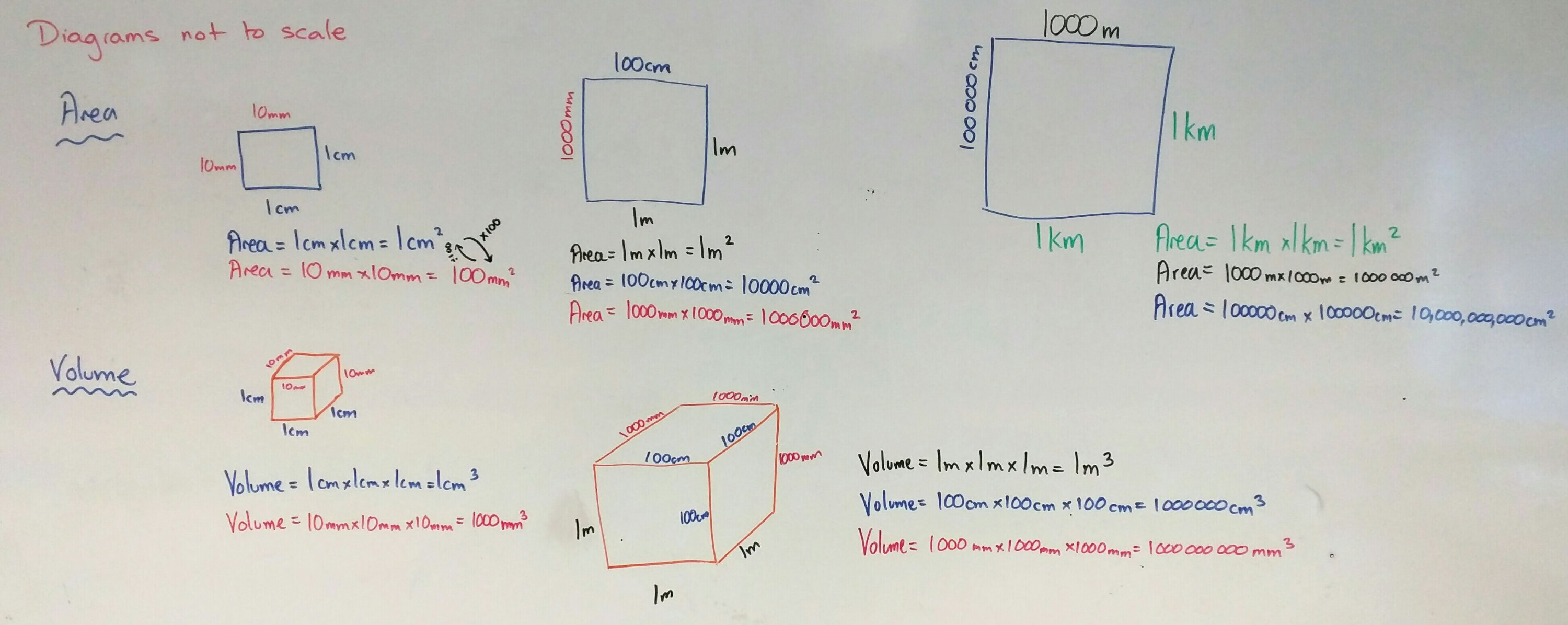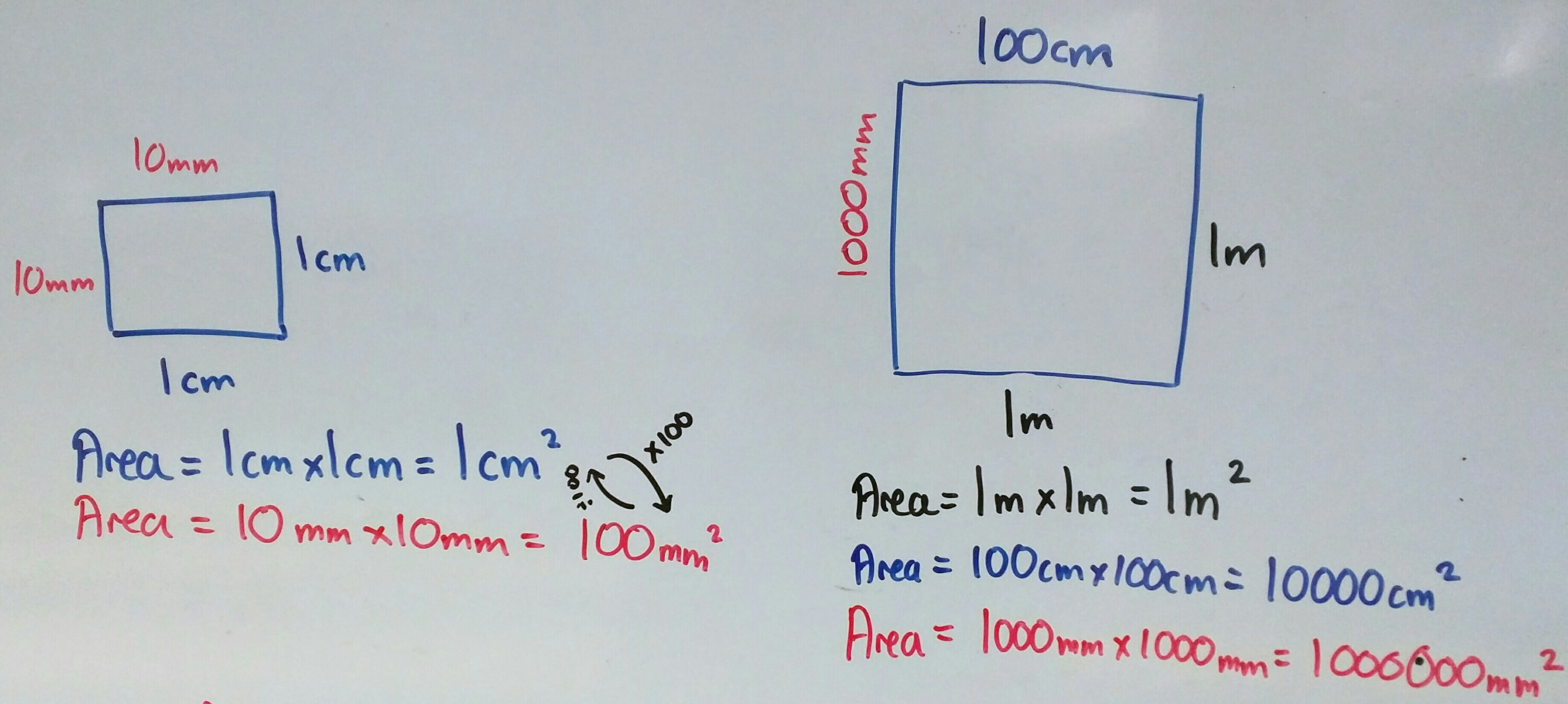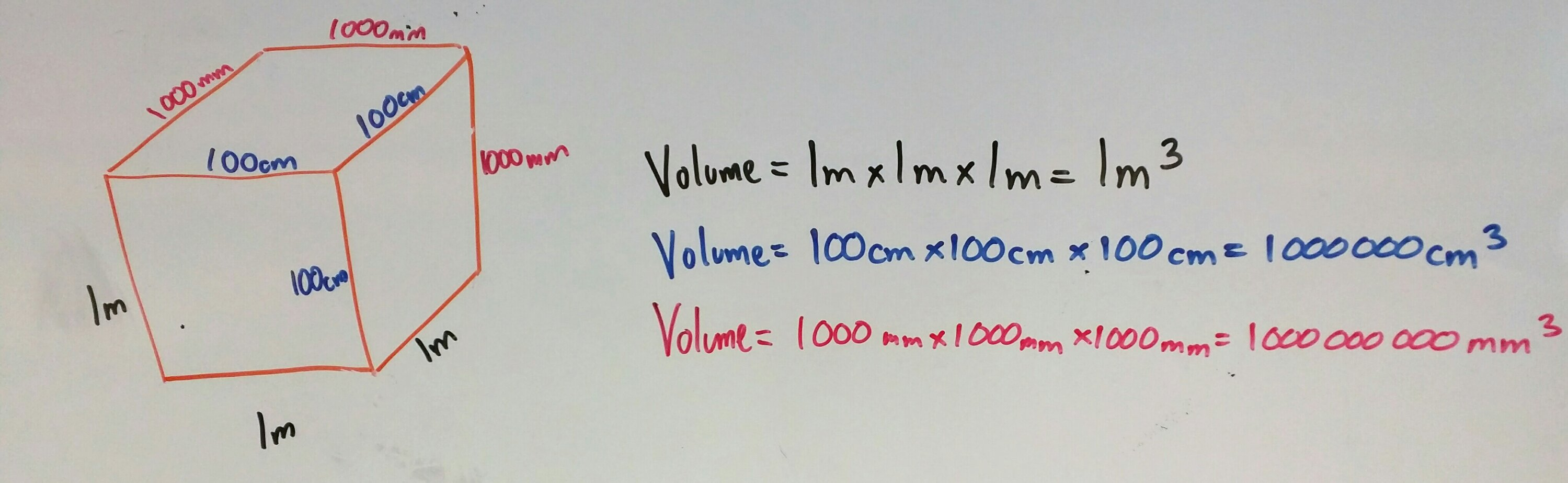# 346. Area & Volume conversion

This is a quick post on how I teach metric unit conversion for area and volume. All you need is a big whiteboard and coloured board pens.Start by stressing that all diagrams are not to scale/accurate.

Two colours

1. Draw a square on the board
2. Pen colour 1: Label it as 1cm
3. What is the area? Show the calculation
4. What is 1cm in mm?
5. Pen colour 2: Label it as 10mm
6. What is the area in mm? Show the calculation
7. What is the scale factor between the sides? the area? why?Three colours

1. Draw a square on the board
2. Pen colour 3: Label it as 1m
3. What is the area? Show the calculation
4. What is 1m in cm?
5. Pen colour 2: Label it as 100cm
6. What is the area in cm? Show the calculation
7. What is the scale factor between the sides? the area? why?
8. Repeat in pen colour 1 for mm

Four colours

Well not actually four colours – pens 2,3 & 4 only. Repeat the process for kilometres to metres and centimetres.

Volume – same process, just three dimensionsWhy all the colours?

By coding each unit of measurement with a colour students can see the progression of the calculations and the links between area/volume and scale factor. After all, an okay mathematician can reproduce memorised facts, but a great mathematician doesn’t need to memorise – they understand where the calculations came from.